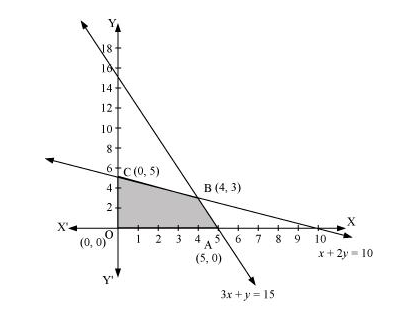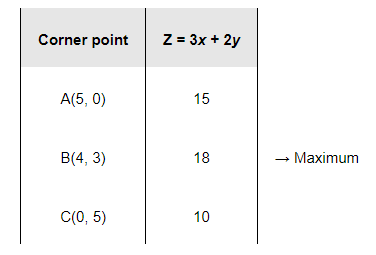# Maximise

Question:

Maximise $Z=3 x+2 y$

subject to $x+2 y \leq 10,3 x+y \leq 15, x, y \geq 0$.

Solution:

The feasible region determined by the constraints, $x+2 y \leq 10,3 x+y \leq 15, x \geq 0$, and $y \geq 0$, is as follows.The corner points of the feasible region are A (5, 0), B (4, 3), and C (0, 5).

The values of Z at these corner points are as follows.Therefore, the maximum value of Z is 18 at the point (4, 3).